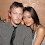## Tuesday, January 26, 2016

### 26 33 42 67 223 | Google Doodle Tribute to Scottish TV Inventor, John Logie Baird

John = 1+6+8+5 = 20
Logie = 3+6+7+9+5 = 30
Baird = 2+1+9+9+4 = 25
John Logie Baird = 75

John = 10+15+8+14 = 47
Logie = 12+15+7+9+5 = 48
Baird = 2+1+9+18+4 = 34
John Logie Baird = 129

8/14/1888 = 8+14+18+88 = 128
8/14/1888 = 8+14+(1+8+8+8) = 47
8/14/1888 = 8+1+4+1+8+8+8 = 38
8/14/88 = 8+14+88 = 110

6/14/1946 = 6+14+19+46 = 85
6/14/1946 = 6+14+(1+9+4+6) = 40
6/14/1946 = 6+1+4+1+9+4+6 = 31
6/14/46 = 6+14+46 = 66

Notice that the man died at age 67, two months shy of his 68th birthday.

Blood Sacrifice = 67
Freemasonry = 67

Notice that he was also Scottish, as in 'Scottish Rite Freemasonry'.

Mason = 4+1+1+6+5 = 17/26

I emphasis the '26' in Mason, because the first working TV was demonstrated on the 26th day of the year 1926.  Keep in mind that '26' is the God number, and it goes without saying, the god of this world we now live in, is largely the TV.

TV = 20+22 = 42 (Freemason = 42)
TV = 24, or 222
T = 20 = 2
V = 22 = 4

Notice the initials of the inventor, JLB.

JLB = 10+12+2 = 24 (TV = 24)

I love the detail about the BBC, or 223, ranking him as the 44th greatest Briton of all time.

Briton = 2+9+9+2+6+5 = 33
Masonic = 223 (Jewish Gematria)

https://en.wikipedia.org/wiki/John_Logie_Baird

1.This comment has been removed by the author.

2.This comment has been removed by the author.

3.8/14/1888 - 6/14/1946
8/14 = date Georgia Guidestones 8/14/2016
1888 = 888 = Donald J Trump (Jew)
6/14/1946 = birthdate Trump
1888 - 2016 = 130 year = 13 / 67 = 6+7 = 13
6/14 - 8/14 = 62 days / Mason = 62
Mechanical Television = 199 = 46th prime number / 1946 / Sacrifice = 46 (Red)
Google Doodle = 116 = 911
Master Mind = 116 behind the Master Plan = 119 = 911
Master Mind = 44 (Red) / Kill = 44 / Master Plan = 38 (Red) / Death = 38
Google Doodle = 62 (Red) / Mason = 62

4.5 years ago I had warts, I was treated with some liquid applied to the warts they continued to grow and spread... The next 2 doctors did laser surgery to remove them. 1 year after the surgery, they grew back close to where the 1st ones were' so I was finally told it was hpv. I have had it for very long time, I contract it from my cheated boyfriend and I found out he was also infected and I end up the relationship between us. the warts was so embarrasses because it started spreading all over I have be dealing with this things for very long time the last treatment I take was About 2 years ago I applied natural treatment from Dr onokun herbal cure, a week after applying the treatment all the warts was gone. it's now 2 years and some months I don't have single wart or any symptoms of hpv. wow"" it's great, Dr onokun has finally cured me. Anyone living with hpv contact Dr onokun for natural treatment.